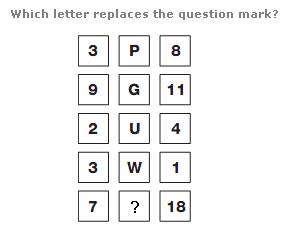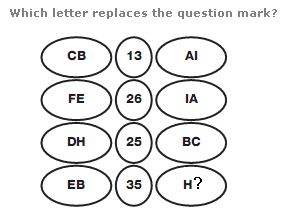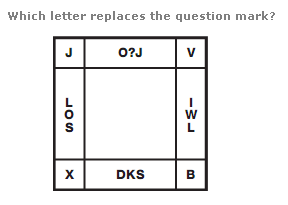# Puzzles - Logical puzzles

### Exercise :: Logical puzzlesAnswer : B Explanation : In each row of the diagram, the reverse alphabetical value of the central letter equals the sum of the left and right hand digits.

View Answer & ExplanationAnswer : G Explanation : Convert each letter to its numerical value, and read each pair of values as 2 digit numbers. In each row, the number in the centre equals the difference between the 2 digit values on the left and right.

View Answer & ExplanationAnswer : Y Explanation : Start in the top left hand corner and move anti clockwise around the perimeter of the square. Letters are written in alphabetical order, skipping 1 letter, then 2 letters, then 3 etc.

View Answer & Explanation

#### Current Affairs 2019

Interview Questions and Answers